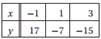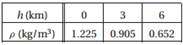Create an Account

Home / Questions / 1 Use Neville s method to determine the equation of the quadratic that passes through the...

1 Use Neville s method to determine the equation of the quadratic that passes through the points 2 The density of air ρ varies with elevation h in the following manner Express ρh as a

1. Use Neville’s method to determine the equation of the quadratic that passes through the points2. The density of air ρ varies with elevation h in the following manner:Express ρ(h) as a quadratic function using Lagrange’s method.

Jul 27 2020 View more View LessSubscribe To Get Solution